Next: 7.2 CMOS Inverter Up: 7. Case Studies Previous: 7. Case Studies

Subsections

# 7.1 Power MOS Devices

Since the invention of semiconductor devices based on the bipolar technology, such as the bipolar transistor (BJT) and the thyristor, strong efforts have been made to increase the power handling capability of these devices in order to extend their applications. As the CMOS technology gained importance and process technology in the field of integrated circuits was surpassing the development of bipolar technology used for power devices new concepts were sought. In the 1970's the power MOSFET was introduced commercially and it was now possible to use the steady progress in CMOS technology also for the development of improved power devices such as the combined MOS/bipolar power devices in the 1980's.

The advantages of power devices based on MOS technology compared to bipolar technology are manifold:

• High input impedance due to the insulated gate contact.
• High switching speed as in contrast to BJTs. The n-channel power MOSFET is operating with electron transport which is inherently faster than the combined electron and hole transport the BJTs rely on.
• As the forward voltage drop in BJTs decreases with increasing temperature they suffer from thermal runaway. Using parallel devices would lead to the concentration of the current on a single device. Power MOSFETs have an increasing forward voltage drop with increasing temperatures and the current is automatically distributed evenly between parallel devices.
• They can be integrated with low voltage circuitry in a monolithic way.
• Larger safe operating area.

## 7.1.1 LDMOSFET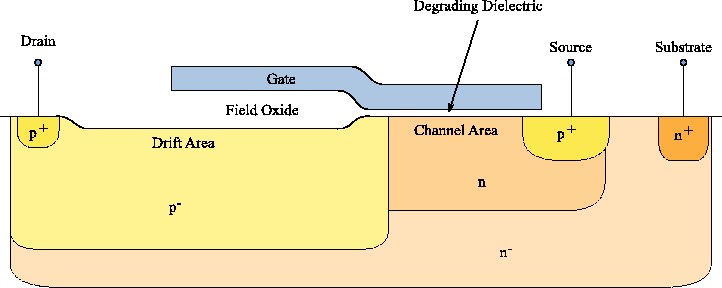For the monolithic integration with low voltage circuitry the lateral double-diffused MOSFET (LDMOSFET) is the preferred device. Figure 7.1 gives a schematic of the device structure.

The channel of the LDMOSFET is formed by two lateral diffusion steps. First the channel doping is implanted in the source region. A following lateral diffusion step moves the dopants under the gate electrode. Then the source doping is implanted in the source region. By means of a second diffusion step the effective channel length can be adjusted. The big advantage is that the channel length is not directly dependent on the feature size of the process technology.

The geometry used for device simulation in this section has been generated using process simulation tools. The maximum voltages for this device are specified asV and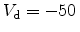V with the other terminals grounded. Figure 7.2 depicts the distribution of the electrostatic potential within the device at these conditions. It can be seen that the major voltage drop between source and drain occurs in the drift area of the device. The typical NBT stress conditions are with zero drain voltage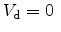V. At this bias condition, the potential at the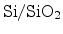interface is nearly constant throughout the device (Figure 7.3).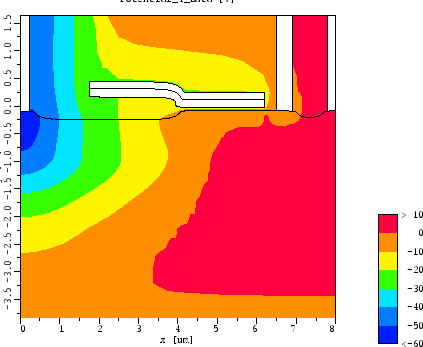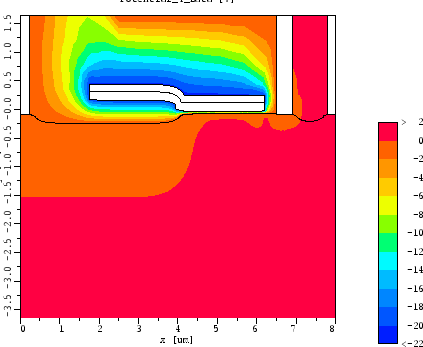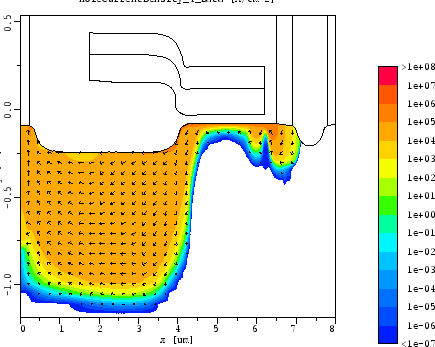Figure 7.4 gives the hole current density of the turned on transistor. In the channel area the current path is very close to the gate oxide, while in the drift region the current flow is deep in the substrate. This shows very well that the state of the silicon/dielectric interface is of highest importance in the gate region, while degradation in the thick oxide above the drift region will have less impact.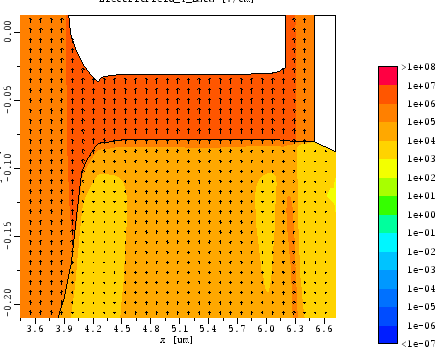Figure 7.5 shows the electric field across the gate dielectric at NBT stress conditions. The field is very homogeneous.

Figure 7.6 gives one-dimensional plots of the electric field and the hole concentration at theinterface. These quantities are of key interest for the NBTI model as proposed in Section 6.6. Because of their even distribution it is reasonable to model gate dielectric degradation due to field dependent degradation mechanisms in one spatial dimension.

Figure 7.6: The magnitude of the (a) electric field and the (b) hole concentration at theinterface. At NBT stress conditions (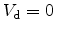V) both quantities are very uniform in the channel area.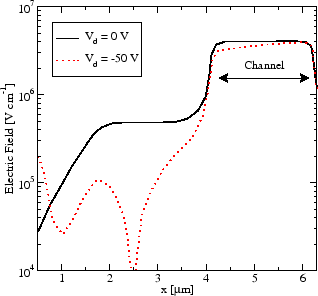Electric field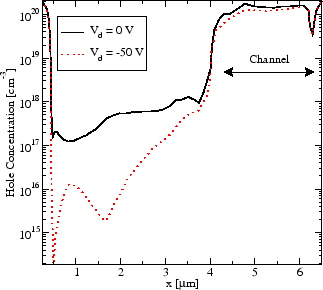Hole concentration

## 7.1.2 Parameter Extraction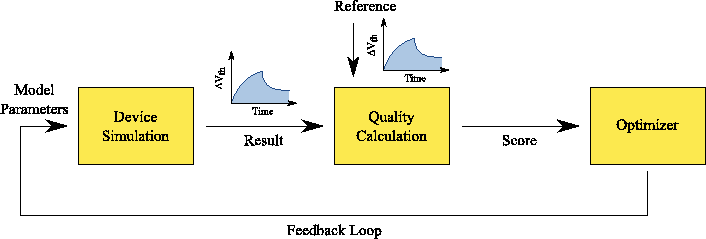The results from Section 7.1.1 propose very uniform conditions along the gate dielectric of LDMOSFETs at NBT stress conditions. The electric field, the hole concentration, and of course the oxide thickness are almost constant in both lateral dimensions. It is therefore possible to calibrate the NBTI model using a simplified device geometry.

For calibration of the NBTI model parameters a conventional p-channel transistor with large gate area has been processed. The oxide of this calibration structure is the same as in the original LDMOSFET. For simulation an identical geometry has been generated.

The procedure to extract the model parameters is schematically depicted in Figure 7.7. After setting up the device geometry an initial set of parameters must be specified. For each parameter a range has to be provided in which it can be adjusted by the optimizer. The duration of the whole optimization process is strongly dependent on these values, so care should be taken to find initial values as close as possible to the optimal calibration point.

In the next step the optimization loop can be started. The first device simulation is performed using the initial set of parameters. The resulting output is evaluated by comparing it to the measurement data. This comparison must result in a single number, the score of the simulation. It is calculated by comparing the simulation output to the measurement data. To generate a single number from the output curves a least square fitting'' criterion was used. Here, the sum of the squares of the offsets is calculated resulting in a score for the fit. A lower score means less offset between measurement and simulation and, thus, a better calibration.

From the score the optimizer generates a new set of model parameters which are then used for the next simulation run. A new score is obtained and this loop continues until the optimizer finds a minimum.

This calibration was first performed for the threshold voltage shift at 175^C. To calibrate the temperature dependence a second calibration run was performed for 100^C using the Arrhenius activation energies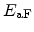,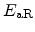, and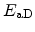as calibration parameters. Table 7.1 gives the NBTI model parameters found for the given device structure. Their definition is given in Section 6.6.

Table 7.1: Calibration results.
 Parameter Value Unit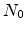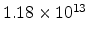cm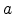2 1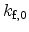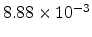s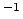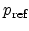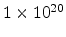cm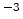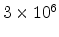V cm0.2238 eV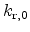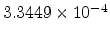cm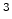s-0.0938 eV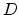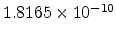cm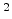s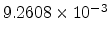eV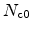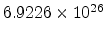cm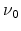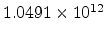s## 7.1.3 Measurements and Simulation Results

The device was stressed with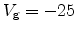V for 1000 seconds and in the following the gate stress was relaxed for another 1000 seconds. This procedure was performed at 100, 125, 150, and 175^C. To determine the threshold voltage shift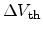, the stress was interrupted for two seconds at each measurement point to perform a gate voltage sweep from 0V to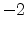V (Section 6.3.1). During this period relaxation can be observed. Thus, it is crucial to include the recovery process in the simulation.

For the comparison of measurements to simulation results the complete dynamics of degradation and annealing during the measurement intervals have to be taken into account. The simulation run was therefore set-up to exactly mimic the measurement. Here, also the threshold voltage was extracted using a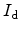/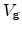sweep to allow relaxation in the same way as in the measurements.

The calibration using the extended model (Section 6.6) shows excellent agreement with measurement data for a wide range of temperatures (Figure 7.8), which can be achieved using a single set of model parameters (Table 7.1).

The pure reaction-diffusion model, in contrast, cannot reproduce the measurement data, as shown in Figure 7.9. In the stress phase a power law slope of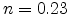under-estimates the measured slope of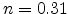. In the relaxation phase the amount of annealing is completely over-estimated.

The inability to calibrate the RD model to the measurement data emphasizes the importance to correctly model the dispersive hydrogen transport in the dielectric. Only the extended model (Section 6.6) is capable of correctly reproducing the data.

Figure 7.8: Result of the calibration process. Very good agreement is found with measurement data using the extended model (Section 6.6). The slope ofduring the stress phase is very well matched with the trap-controlled transport model and the agreement in the relaxation phase is excellent.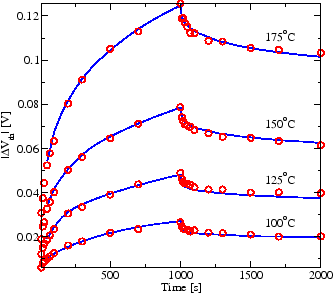Linear scale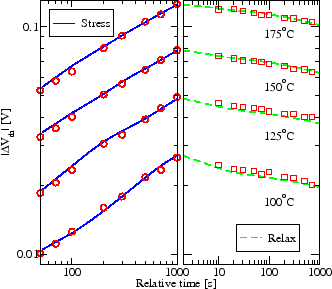Logarithmic scale

Figure 7.9: The pure reaction-diffusion model without dispersion. This model obtains a slope ofand completely fails to reproduce the measurement data. Especially the annealing in the relaxation phase is drastically over-estimated.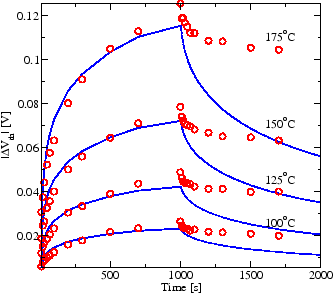Linear scale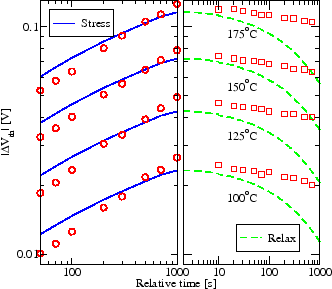Logarithmic scaleNext: 7.2 CMOS Inverter Up: 7. Case Studies Previous: 7. Case Studies

R. Entner: Modeling and Simulation of Negative Bias Temperature Instability